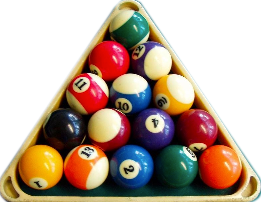【每日一题0104】台球金字塔还记得台球桌上的摆球三角形吗？如果需要摆满经典的5层则需要15颗球。如果拥有3颗球，则可以摆出2层结构。请按照这个规律，编写一个函数，接收一个表示球数num，返回它们可以构成的三角形层的层数。def solution(num: int) -> int:
# pass

assert solution(1) == 1
assert solution(4) == 2
assert solution(20) == 5
assert solution(2211) == 66
assert solution(9999) == 140
def solution14(num:int):
sum=0
for i in range(0,num):
sum+=i
if num==1:
return 1
elif sum == num:
return i
elif sum > num:
return i-1

assert solution14(1) == 1
assert solution14(4) == 2
assert solution14(20) == 5
assert solution14(2211) == 66
assert solution14(9999) == 140
def solution(num: int) -> int:
n = 1
sum = 0
while True:
sum += n
if sum == num:
return n
elif sum < num:
n += 1
else:
return n-1

assert solution(1) == 1
assert solution(4) == 2
assert solution(20) == 5
assert solution(2211) == 66
assert solution(9999) == 140
def solution(num: int) -> int:
# pass
for n in range(0, num+1):
if num == ((1 + n) * n / 2):
return n
elif num < ((1 + n) * n / 2):
return n - 1

assert solution(1) == 1
assert solution(4) == 2
assert solution(20) == 5
assert solution(2211) == 66
assert solution(9999) == 140
1 Like
def solution(num: int) -> int:
return 1 if num == 1 else [i - 1 for i in range(num) if i * (i + 1) > 2 * num]

assert solution(1) == 1
assert solution(4) == 2
assert solution(20) == 5
assert solution(2211) == 66
assert solution(9999) == 140
1 Like
def sum(n):
res = 0
for i in range(1, n + 1):
res += i
return res

def solution(num: int) -> int:
layer = int((2 * num + .25) ** .5 - .5)
while layer > 0:
if sum(layer) <= num < sum(layer + 1):
return layer
else:
layer -= 1
pass

assert solution(1) == 1
assert solution(4) == 2
assert solution(20) == 5
assert solution(2211) == 66
assert solution(9999) == 140

[/quote]

def solution(num: int) -> int:
i = 1
while num-i >= 0:
num = num-i
i += 1
return i - 1

assert solution(1) == 1
assert solution(4) == 2
assert solution(20) == 5
assert solution(2211) == 66
assert solution(9999) == 140
1 Like
def solution(num: int) -> int:
if num<1: return 0
level,result = 0,0
while result<num:
level+=1
result += level
return level if result == num else level-1

assert solution(1) == 1
assert solution(4) == 2
assert solution(20) == 5
assert solution(2211) == 66
assert solution(9999) == 140

assert solution(2) == 1
assert solution(0) == 0
assert solution(-1) == 0